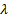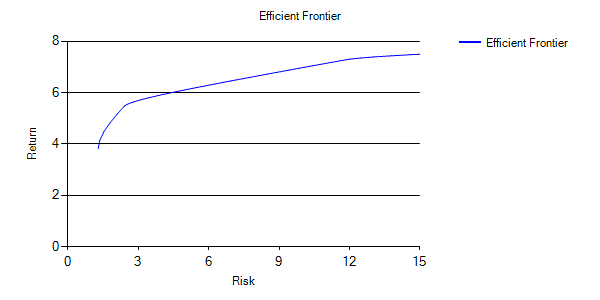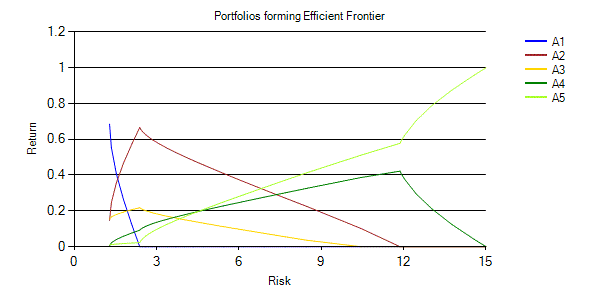/

### Extreme Events – Specimen Question A.5.1(a) – Answer/Hints

Q. Plot the efficient frontier and the asset mixes making up the points along the efficient frontier, assuming that risk-free is to be equated with zero volatility of return and that no non-negative holdings are allowed for any asset category.

The efficient frontier can be found using standard constrained quadratic optimisation techniques, constrained quadratic optimisation techniques and then plotting the results.

This can be done using the Solver add-in that comes as standard with Microsoft Excel, but rather simpler (in our opinion) is to make use of the Nematrian online toolkit or an equivalent. The Nematrian toolkit provides three different tools, each one of which can be used to solve this particular problem:

(a)    The asset mix (and corresponding risk and expected return) corresponding to a single point along the efficient frontier (i.e. for a specific, i.e. risk-reward trade-off parameter) can be found interactively using Nematrian’s Example Quadratic Portfolio Optimiser page.

(b)    In this case we want to plot the efficient frontier, i.e. we want the risk and expected returns for a range of points along the efficient frontier (and we also want to plot the corresponding asset mixes). We could run (a) for several different efficient frontiers, but this would be quite laborious. Simpler than (a) (particularly if you are likely to carry out several similar exercises) may be to call the corresponding Nematrian MnConstrainedQuadraticPortfolioOptimiser web service function many times using VBA.

(c)     Perhaps best in this instance is to piggy-back off of the Nematrian website’s SmartChart facility and to use the (standard) Nematrian web function plotting equivalents to (b), i.e. MnPlotQuadraticEfficientFrontier and MnPlotQuadraticEfficientPortfolios respectively. The web function itself returns a string corresponding to the SmartCode of a suitable Smart Chart. The interactive variant returns an entire (temporary) Nematrian SmartChart (which incorporates this SmartCode).

Even simpler, if it exists, is to use a previously established spreadsheet that embeds the relevant Nematrian web functions. For this particular purpose one does exist and can be found here. Other spreadsheets that simultaneously illustrate a selection of related Nematrian web functions can be found here.

Permanent SmartCharts corresponding to (c) are shown below. To equate risk free with zero volatility the minimum risk portfolio should be input as {0,0,0,0,0}. To bar non-negative holdings, place a lower limit of zero on each holding, i.e. the Lower Bounds should be input as {0,0,0,0,0}. Asset weights must add to unity, which can be achieved by suitable choice of Constraint Matrix, Constraint Limits and Constraint Types. A full symmetric correlation matrix needs to be input into Forecast Correlations, i.e. here:

 A1 A2 A3 A4 A5 A1 1 0.4 -0.6 0.0 -0.4 A2 0.4 1 -0.5 -0.4 -0.4 A3 -0.6 -0.5 1 0.2 0.6 A4 0.0 -0.4 0.2 1 0.3 A5 -0.4 -0.4 0.6 0.3 1

Some playing around with the input lambda values may be needed to get a set that span the entire range of efficient portfolios. For the charts below we have used the following input values of lambda:

 0.1 1 2 3 4 5 7 10 12 14 16 18 20 25 30 35 40 45 50 60 70 80 90 100 125 150 175 200 300 400 500 600 700 900

Efficient frontier:Portfolios forming the efficient frontier